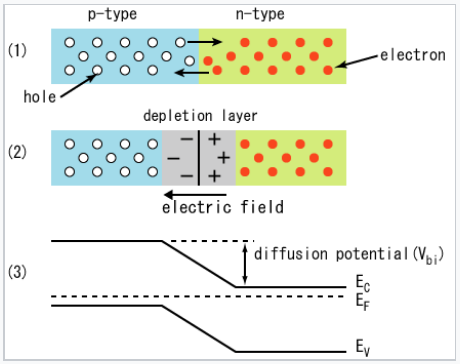# 9.10: Semiconductor p-n Junctions

$$\newcommand{\vecs}{\overset { \rightharpoonup} {\mathbf{#1}} }$$ $$\newcommand{\vecd}{\overset{-\!-\!\rightharpoonup}{\vphantom{a}\smash {#1}}}$$$$\newcommand{\id}{\mathrm{id}}$$ $$\newcommand{\Span}{\mathrm{span}}$$ $$\newcommand{\kernel}{\mathrm{null}\,}$$ $$\newcommand{\range}{\mathrm{range}\,}$$ $$\newcommand{\RealPart}{\mathrm{Re}}$$ $$\newcommand{\ImaginaryPart}{\mathrm{Im}}$$ $$\newcommand{\Argument}{\mathrm{Arg}}$$ $$\newcommand{\norm}{\| #1 \|}$$ $$\newcommand{\inner}{\langle #1, #2 \rangle}$$ $$\newcommand{\Span}{\mathrm{span}}$$ $$\newcommand{\id}{\mathrm{id}}$$ $$\newcommand{\Span}{\mathrm{span}}$$ $$\newcommand{\kernel}{\mathrm{null}\,}$$ $$\newcommand{\range}{\mathrm{range}\,}$$ $$\newcommand{\RealPart}{\mathrm{Re}}$$ $$\newcommand{\ImaginaryPart}{\mathrm{Im}}$$ $$\newcommand{\Argument}{\mathrm{Arg}}$$ $$\newcommand{\norm}{\| #1 \|}$$ $$\newcommand{\inner}{\langle #1, #2 \rangle}$$ $$\newcommand{\Span}{\mathrm{span}}$$

Semiconductor p-n junctions are important in many kinds of electronic devices, including diodes, transistors, light-emitting diodes, and photovoltaic cells. To understand the operation of these devices, we first need to look at what happens to electrons and holes when we bring p-type and n-type semiconductors together. At the junction between the two materials, mobile electrons and holes annihilate each other, leaving behind the fixed + and - charges of the electron donor and electron acceptor dopants, respectively. For example, on the n-side of a silicon p-n junction, the positively charged dopants are P+ ions and on the p-side the negatively charged dopants are B-. The presence of these uncompensated electrical charges creates an electric field, the built-in field of the p-n junction. The region that contains these charges (and a very low density of mobile electrons or holes) is called the depletion region.

The electric field, which is created in the depletion region by electron-hole recombination, repels both the electrons (on the n-side) and holes (on the p-side) away from the junction. The concentration gradient of electrons and holes, however, tends to move them in the opposite direction by diffusion. At equilibrium, the flux of mobile carriers is zero because the field-driven migration flux is equal and opposite to the concentration-driven diffusion flux.When p-type and n-type semiconductors are joined, electrons and holes are annihilated at the interface, leaving a depletion region that contains positively and negatively charged donor and acceptor atoms, respectively. At equilibrium, the Fermi level (EF) is uniform throughout the junction. EF lies just above the valence band on the p-type side of the junction and just below the conduction band on the n-type side.

The width of the depletion layer depends on the screening length in the semiconductor, which in turn depends on the dopant density. At high doping levels, the depletion layer is narrow (tens of nanometers across), whereas at low doping density it can be as thick as 1 µm. The depletion region is the only place where the electric field is nonzero, and the only place where the bands bend. Elsewhere in the semiconductor the field is zero and the bands are flat.

In the middle of p-n junction, the Fermi level energy, EF, is halfway between the valence band, VB, and the conduction band, CB, and the semiconductor is intrinsic (n = p = ni)

9.10: Semiconductor p-n Junctions is shared under a CC BY-SA license and was authored, remixed, and/or curated by LibreTexts.